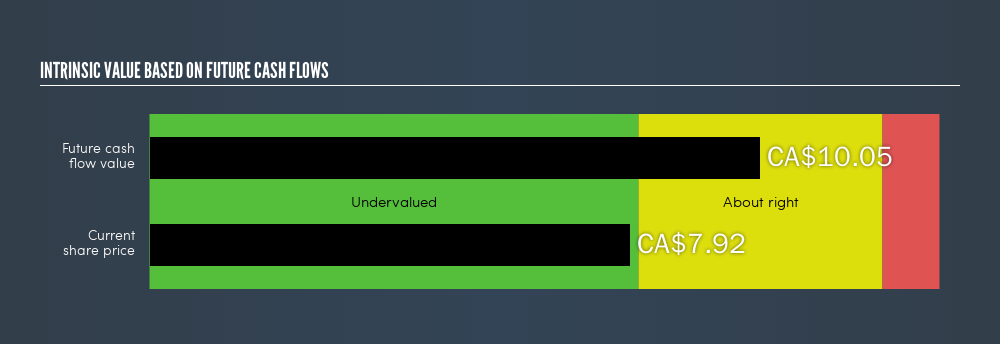latest

Investors Are Undervaluing iAnthus Capital Holdings, Inc. (CNSX:IAN) By 21.21%

Want to participate in a short research study? Help shape the future of investing tools and receive a \$20 prize!

In this article I am going to calculate the intrinsic value of iAnthus Capital Holdings, Inc. (CNSX:IAN) by taking the expected future cash flows and discounting them to their present value. I will use the discounted cash flows (DCF) model. It may sound complicated, but actually it is quite simple! Anyone interested in learning a bit more about intrinsic value should have a read of the Simply Wall St analysis model. If you are reading this and its not February 2019 then I highly recommend you check out the latest calculation for iAnthus Capital Holdings by following the link below.

Crunching the numbers

I use what is known as a 2-stage model, which simply means we have two different periods of varying growth rates for the company’s cash flows. Generally the first stage is higher growth, and the second stage is a more stable growth phase. In the first stage we need to estimate the cash flows to the business over the next five years. For this I used the consensus of the analysts covering the stock, as you can see below. I then discount the sum of these cash flows to arrive at a present value estimate.

5-year cash flow estimate

 2019 2020 2021 2022 2023 Levered FCF (\$, Millions) \$-134.00 \$29.00 \$162.00 \$229.00 \$319.00 Source Analyst x1 Analyst x1 Analyst x1 Analyst x1 Analyst x1 Present Value Discounted @ 18.05% \$-113.51 \$20.81 \$98.47 \$117.90 \$139.13

Present Value of 5-year Cash Flow (PVCF)= US\$263m

We now need to calculate the Terminal Value, which accounts for all the future cash flows after the five years. The Gordon Growth formula is used to calculate Terminal Value at an annual growth rate equal to the 10-year government bond rate of 1.9%. We discount this to today’s value at a cost of equity of 18.1%.

Terminal Value (TV) = FCF2023 × (1 + g) ÷ (r – g) = US\$319m × (1 + 1.9%) ÷ (18.1% – 1.9%) = US\$2.0b

Present Value of Terminal Value (PVTV) = TV / (1 + r)5 = US\$2.0b ÷ ( 1 + 18.1%)5 = US\$881m

The total value is the sum of cash flows for the next five years and the discounted terminal value, which results in the Total Equity Value, which in this case is US\$1.1b. The last step is to then divide the equity value by the number of shares outstanding. If the stock is an depositary receipt (represents a specified number of shares in a foreign corporation) then we use the equivalent number. This results in an intrinsic value of CA\$10.05. Compared to the current share price of CA\$7.92, the stock is about right, perhaps slightly undervalued at a 21% discount to what it is available for right now.CNSX:IAN Intrinsic value, February 23rd 2019

The assumptions

I’d like to point out that the most important inputs to a discounted cash flow are the discount rate and of course the actual cash flows. If you don’t agree with my result, have a go at the calculation yourself and play with the assumptions. Because we are looking at iAnthus Capital Holdings as potential shareholders, the cost of equity is used as the discount rate, rather than the cost of capital (or weighed average cost of capital, WACC) which accounts for debt. In this calculation I’ve used 18.1%, which is based on a levered beta of 2. This is derived from the Bottom-Up Beta method based on comparable companies, with an imposed limit between 0.8 and 2.0, which is a reasonable range for a stable business.

Next Steps:

Valuation is only one side of the coin in terms of building your investment thesis, and it shouldn’t be the only metric you look at when researching a company. What is the reason for the share price to differ from the intrinsic value? For IAN, I’ve put together three relevant factors you should further examine:

1. Financial Health: Does IAN have a healthy balance sheet? Take a look at our free balance sheet analysis with six simple checks on key factors like leverage and risk.
2. Future Earnings: How does IAN’s growth rate compare to its peers and the wider market? Dig deeper into the analyst consensus number for the upcoming years by interacting with our free analyst growth expectation chart.
3. Other High Quality Alternatives: Are there other high quality stocks you could be holding instead of IAN? Explore our interactive list of high quality stocks to get an idea of what else is out there you may be missing!

PS. Simply Wall St does a DCF calculation for every CA stock every 6 hours, so if you want to find the intrinsic value of any other stock just search here.

We aim to bring you long-term focused research analysis driven by fundamental data. Note that our analysis may not factor in the latest price-sensitive company announcements or qualitative material.

If you spot an error that warrants correction, please contact the editor at editorial-team@simplywallst.com. This article by Simply Wall St is general in nature. It does not constitute a recommendation to buy or sell any stock, and does not take account of your objectives, or your financial situation. Simply Wall St has no position in the stocks mentioned. Thank you for reading.